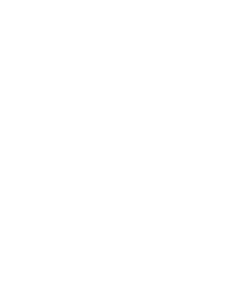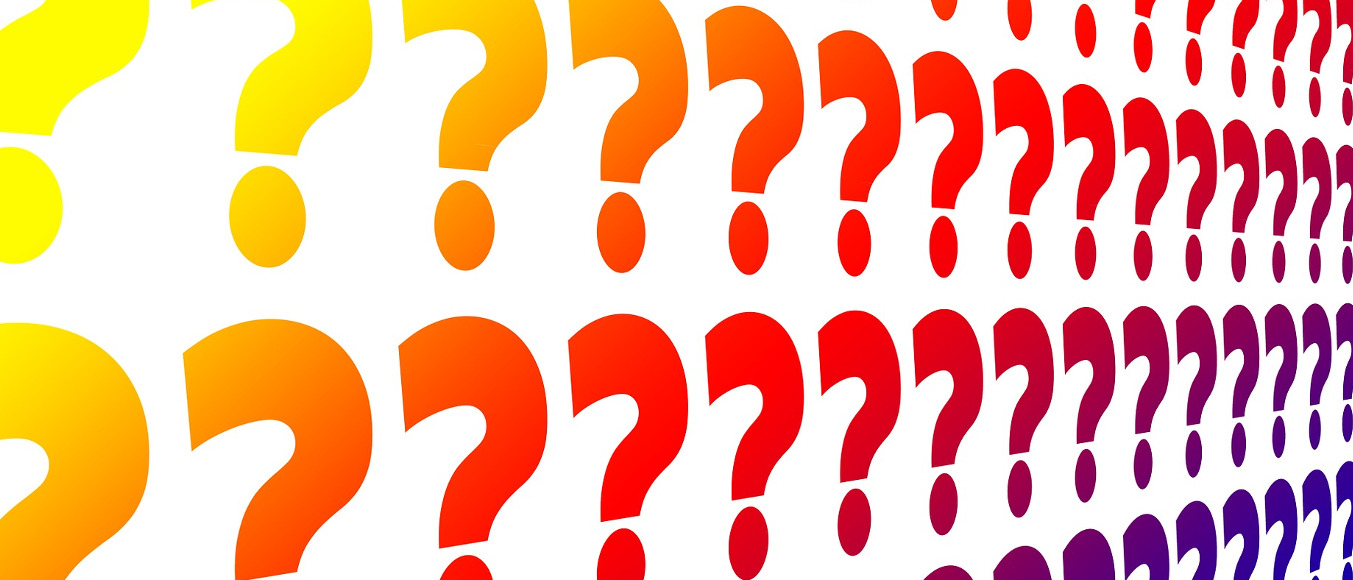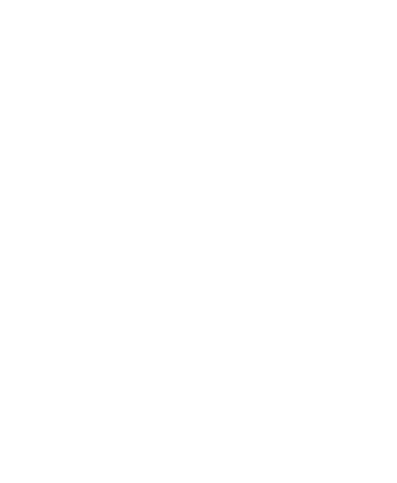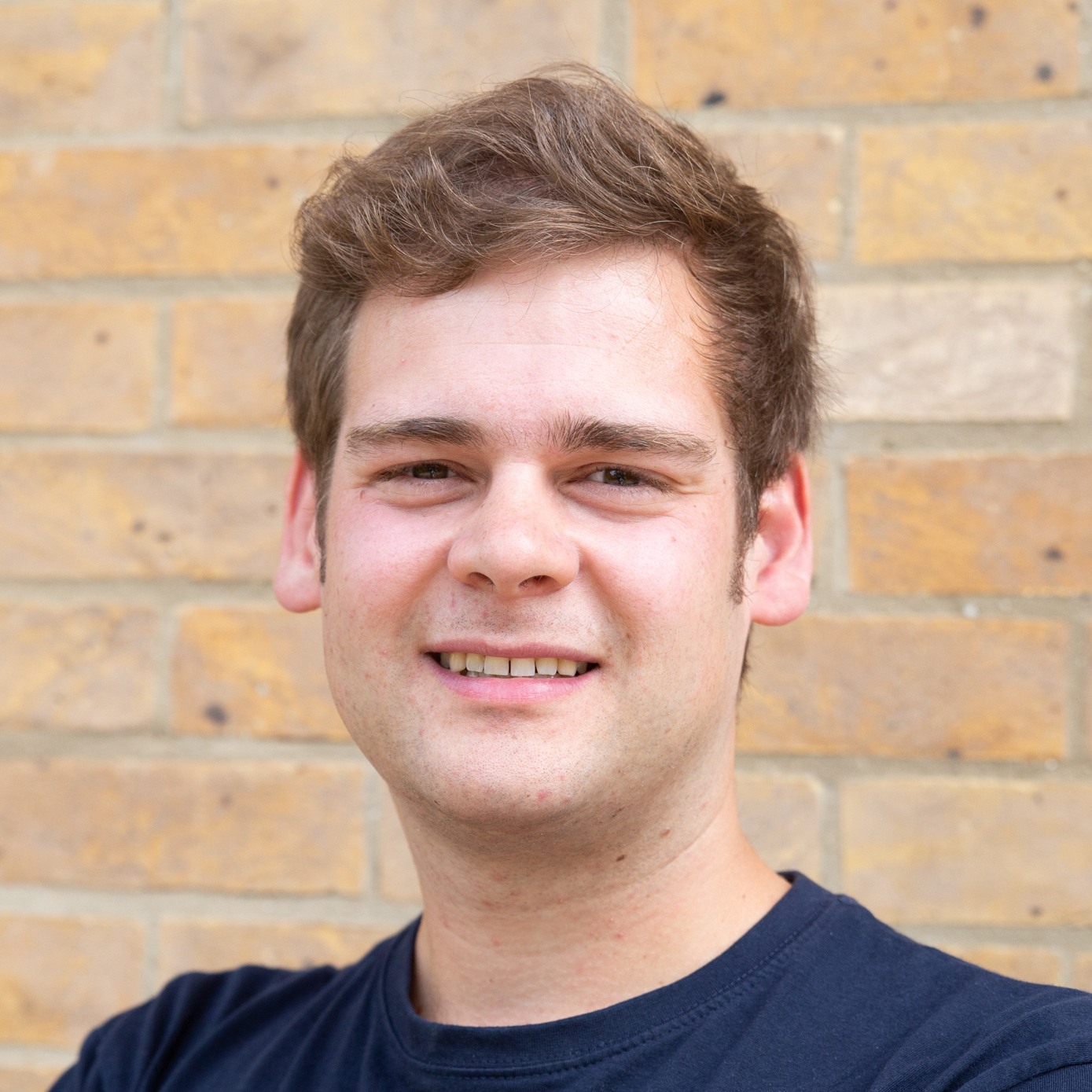# Puzzles, Issue 02

These puzzles appeared in Issue 02 of the magazine.

### Seven Digits

Source: My Best Puzzles in Mathematics by Hubert Phillips
I’m thinking of a number. I’ve squared it. I’ve squared the square. And I’ve multiplied the second square by the original number.

So I now have a number of seven digits whose final digit is a seven.

What was my original number?

### Odd Squares

How many square numbers are there whose digits are all odd?### Folding Tube Maps

Source: mscroggs.co.uk
In How To Make, you will find the instructions for folding a tetrahedron from two tube maps.

The resulting tetrahedron is almost (but not quite) regular.

What ratio would the sides of the tube maps have to be to make a regular tetrahedron?

The answers to these puzzles will be available here from 1 November 2015.These puzzles appeared in Issue 02 of the magazine.

### Seven Digits

Source: My Best Puzzles in Mathematics by Hubert Phillips
I’m thinking of a number. I’ve squared it. I’ve squared the square. And I’ve multiplied the second square by the original number.

So I now have a number of seven digits whose final digit is a seven.

What was my original number?

### Odd Squares

How many square numbers are there whose digits are all odd?### Folding Tube Maps

Source: mscroggs.co.uk
In How To Make, you will find the instructions for folding a tetrahedron from two tube maps.

The resulting tetrahedron is almost (but not quite) regular.

What ratio would the sides of the tube maps have to be to make a regular tetrahedron?

The answers to these puzzles will be available here from 1 November 2015.Matthew Scroggs is a postdoctoral researcher in the Department of Engineering at the University of Cambridge working on finite and boundary element methods. His website, mscroggs.co.uk, is full of maths.
@mscroggs    mscroggs.co.uk    + More articles by Matthew